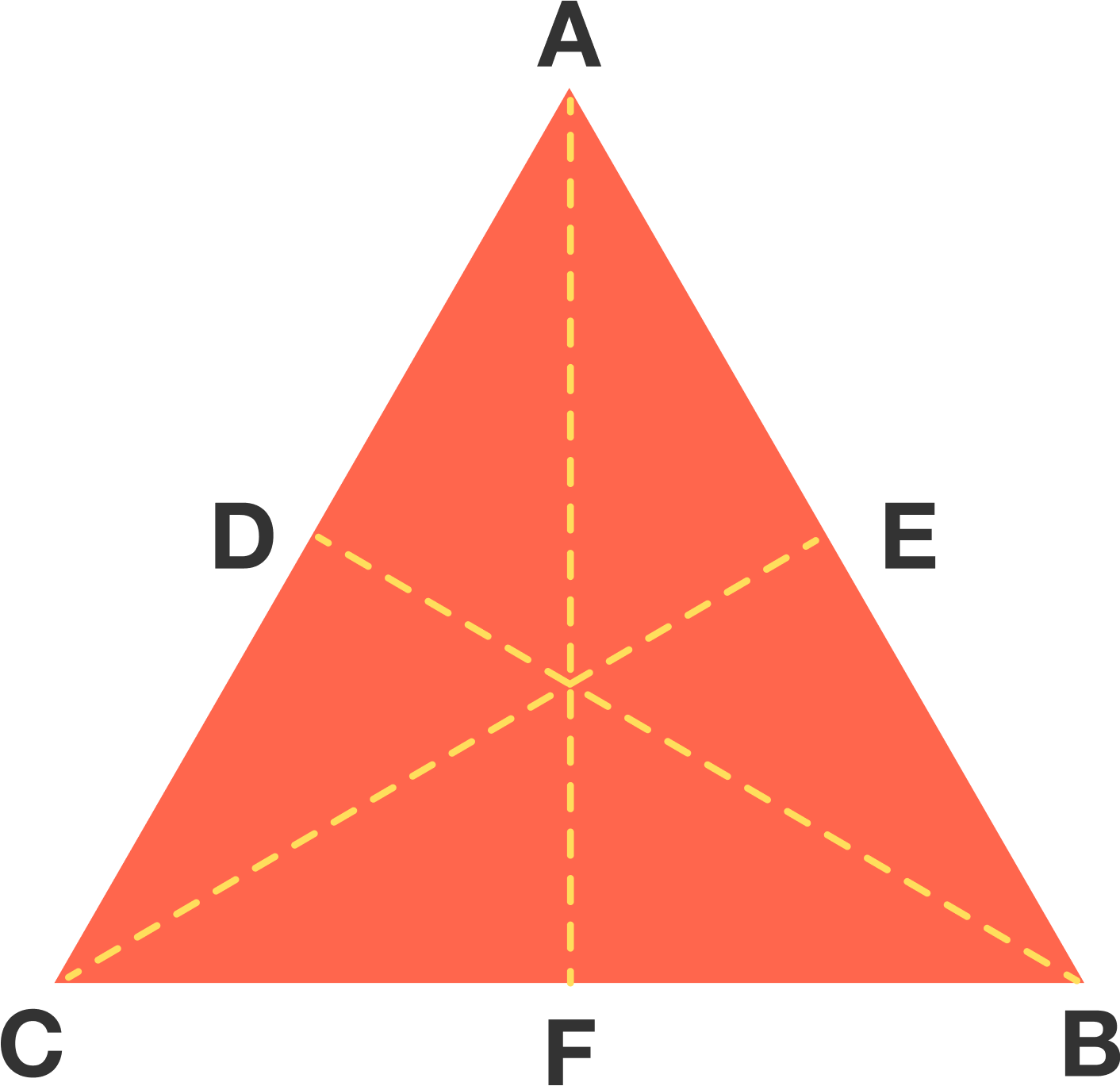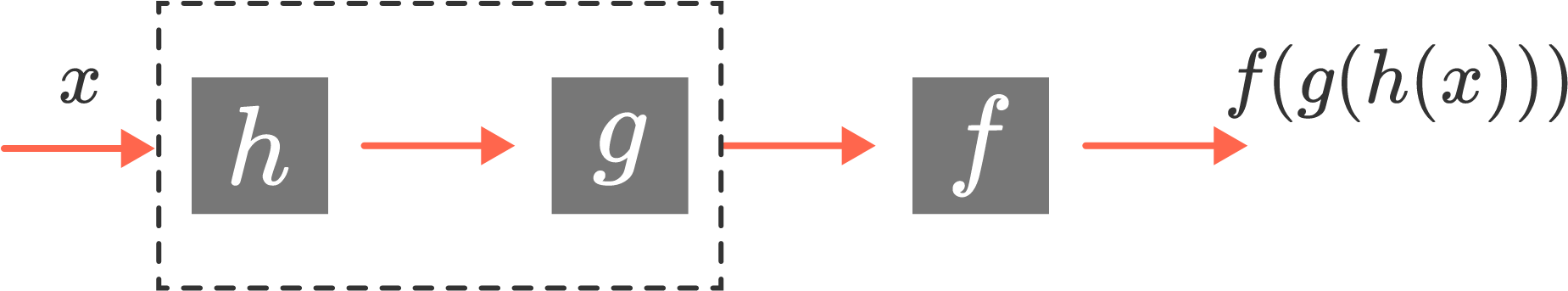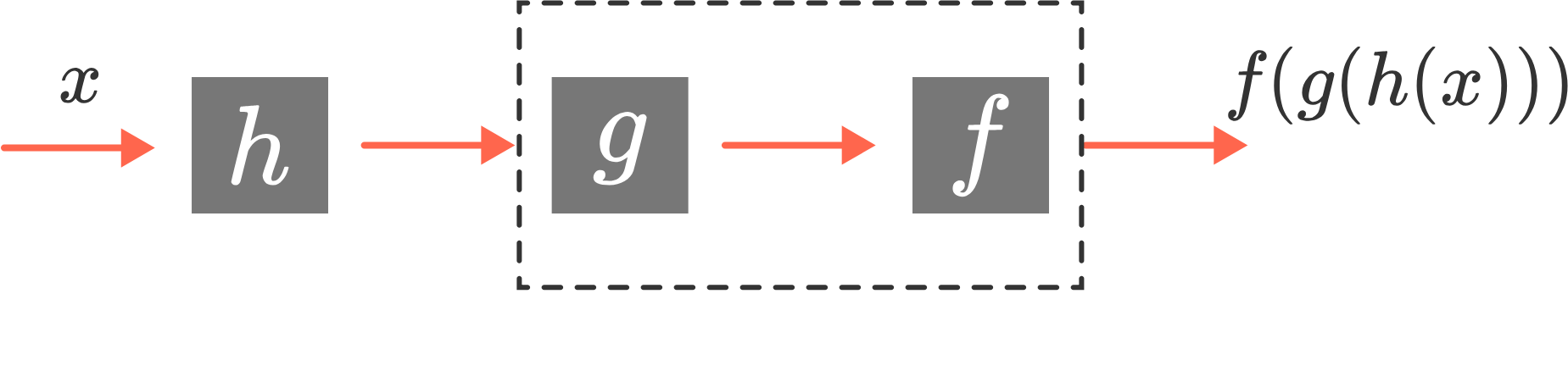### Group Theory

So far, we’ve seen that for a given shape, the set of its symmetries forms an algebraic object, meaning that there is a kind of “multiplication”: Given two symmetries $S$ and $T,$ their product $ST$ is the transformation you get by applying $T$ first, and then $S.$ This corresponds to taking the composition of the transformations $S$ and $T.$

Let’s look at some of the properties these algebraic systems always have, and we’ll use these properties to motivate our definition of a group.

# Group Axioms

Identity

Every collection of symmetries has the identity symmetry $I,$ given by the function $f(x)=x,$ that “does nothing.” So what happens when you multiply $I$ by the other symmetries?

True or False?

$S * I = S$ and $I * S = I$ for all symmetries $S$ of the shape.

# Group Axioms

Inverses

Because the underlying functions are all bijections, they all have inverses. Intuitively, this means that given any symmetry $S$ of a shape, there is a symmetry, called the inverse of $S,$ that “undoes” $S.$ For example, with the equilateral triangle, if $S$ is clockwise rotation by $120^{\circ},$ then the inverse of $S$ is counterclockwise rotation by $120^{\circ}.$

If $T$ is a reflection symmetry of the equilateral triangle, what is the inverse of $T?$

# Group Axioms

Inverses, continued

Suppose $S$ is a symmetry and $T$ is its inverse. If we apply $S$ to an element, how do we express the idea that “$T$ undoes $S$” in multiplication language?

# Group Axioms

Commutativity

One nice property that some algebraic systems have is commutativity. This means that when you perform the operation, the order of the two arguments doesn’t matter. For example, for any two real numbers $x$ and $y$, $x*y = y*x.$ We say that real number multiplication is commutative. Real number addition is also commutative.

But what about the algebraic system of symmetries? Let’s look at the equilateral triangle again. Given any two symmetries $S$ and $T$ of the triangle (that is, where $T$ is no longer necessarily the inverse), does it follow that $S * T = T * S$?# Group Axioms

Associativity

So commutativity doesn’t always hold in our systems of symmetries, and we therefore won’t include it as one of our group axioms.

What about associativity? The associative property in the case of real numbers says that given real numbers $x,$ $y$ and $z,$ we have $(x * y) * z = x * (y * z).$ Does a similar law hold for rigid transformations of a shape?

The answer is yes, because the “multiplication” here is just function composition, and composition is always associative. The reason why is a mathematical argument that’s so simple it’s a little tricky, and we'll explore it next.

# Group Axioms

Here’s the so-simple-it’s-tricky argument for associativity of function composition. (You can skip this page if you like, although it's an interesting argument!)

Imagine we have three functions $f,$ $g$ and $h,$ which we are going to compose together in the order shown below. So we take an input $x$ on the left, and feed it all the way through the system: first we feed it to $h,$ then we feed the result $h(x)$ to $g,$ and then we feed that result $g(h(x))$ to $f$, yielding $f(g(h(x)))$ at the end.To show associativity, we wish to compare $(f \circ g) \circ h$ with $f \circ (g \circ h)$. But $f \circ (g \circ h)$ is the function you get by first doing $g \circ h,$ and then doing $f;$ in other words, by “grouping together” the leftmost two functions into a single box:On the other hand, $(f \circ g) \circ h$ is the function you get by first doing $h$ and then doing $f \circ g;$ in other words, by “grouping together” the rightmost two functions into a single function box:But putting additional boxes around functions doesn’t change the assembly line: in both cases, we take an input $x$ and feed it into $h,$ then the result of that into $g,$ and then the result of that into $f,$ producing $f(g(h(x))).$ Hence $(f \circ g) \circ h$ and $f \circ (g \circ h)$ are the same function, and we’ve proven associativity of function composition!

# Group Axioms

Let’s recap. Given a shape, if we take the set of symmetries of the shape, and consider their composition as a kind of “multiplication,” the following things are true:

(Identity) There is a symmetry $I$ with the property that $I * S = S * I = S$ for all symmetries $S.$

(Inverses) Given any symmetry $S,$ there is a symmetry $T$ such that $S * T = T * S = I.$

(Associativity) Given any symmetries $R,$ $S,$ and $T,$ we have $R * (S * T) = (R * S) * T.$

(We purposely don’t include commutativity, because it doesn’t hold with all symmetries, including ours.)

Nineteenth century mathematicians discovered many examples of algebraic systems satisfying these three axioms, and realized that instead of studying all of these systems separately, we could look at systems that satisfy these axioms in the abstract. This would be like studying all the special cases at the same time. Such a study gives a new perspective on all the special cases. Instead of being among the trees, we can look down on the whole forest from the perspective of a mountaintop.

# Group Axioms

We have finally come to the idea of the group: an abstract object with a “multiplication operation” defined, that satisfies the three axioms. The symmetries we have been looking at are examples of groups, but there are a lot more interesting examples to come, some of them fun and some of them strange. You will see what powerful insight you get by standing on the mountaintop!

# Group Axioms

×